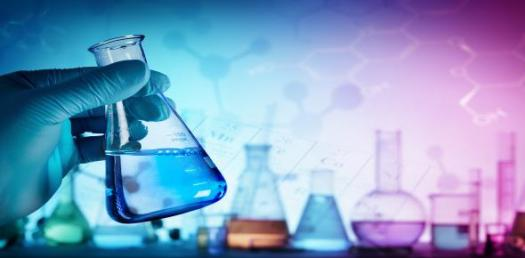# How Much Do You Know About Chemical Kinetics?

10 Questions | Total Attempts: 107SettingsChemical kinetics is an interesting part of chemistry concerned with the rate of chemical process. Research in chemical research is conducted to understand how alterations in chemical reactions affect the progress of the chemical progress. It involves creation and use of mathematical models to make this happen. You already know this? Take this quiz now!

• 1.
Which of these refers to the property of thermodynamic system?
• A.

Entropy

• B.

Heat of reaction

• C.

Enthalpy

• D.

Gibb's free energy

• 2.
Which of these is irrelevant as far as the nature of reactants is concerned?
• A.

Molecules

• B.

Acid

• C.

Bases

• D.

Salts

• 3.
Which of these is not standard, physical state?
• A.

Liquid

• B.

Gas

• C.

Solid

• D.

Metalloid

• 4.
What brings reactants in aqueous solution to contact?
• A.

Thermal motion

• B.

Van der waal forces

• C.

Cohesive force

• D.

• 5.
Which of is less likely to react fast?
• A.

Liquid reactants

• B.

Solid reactants

• C.

Aqueous reactants

• D.

Gaseous reactants

• 6.
Which of these is usually not in solid state?
• A.

Potash

• B.

Camphor

• C.

Hydrochloric acid

• D.

Ice

• 7.
Which of these factors affecting the rate of reaction involve energy?
• A.

Temperature

• B.

Concentration of reactants

• C.

Physical state

• D.

Surface area of reactants

• 8.
What is the standard temperature on which the rate of chemical reaction is based?
• A.

180° C

• B.

273° C

• C.

90° C

• D.

360° C

• 9.
What are proteins that act as catalysts called?
• A.

Enzyme

• B.

Alkaloids

• C.

Base

• D.

Metalloid

• 10.
What is the concentration of oxygen in the atmosphere?
• A.

55%

• B.

21%

• C.

19%

• D.

29%

Related TopicsBack to top